#DAY 21
0

## [Day-21] Wide & Deep 推薦系統實作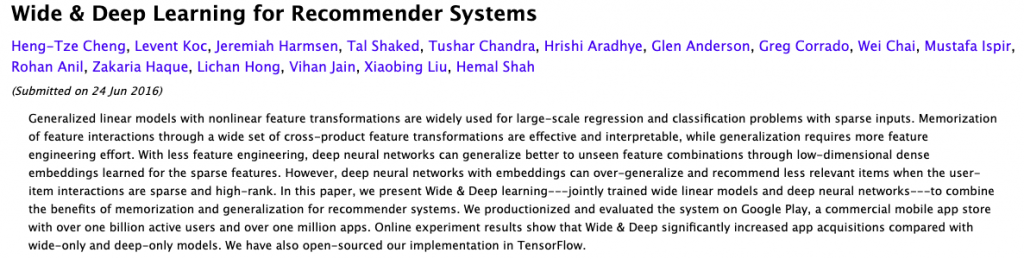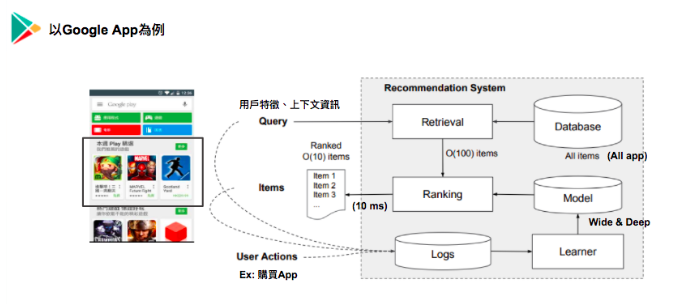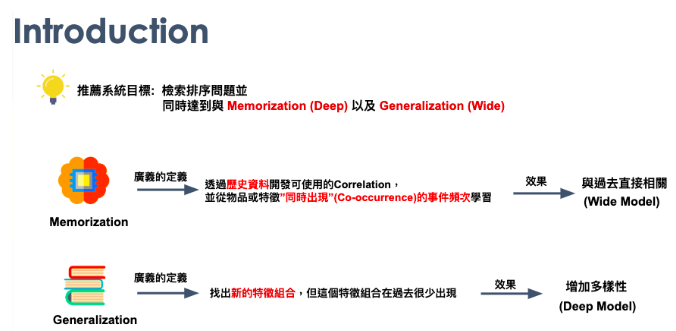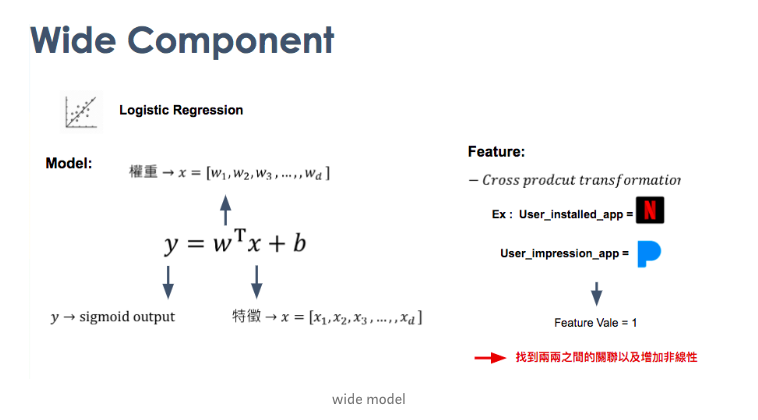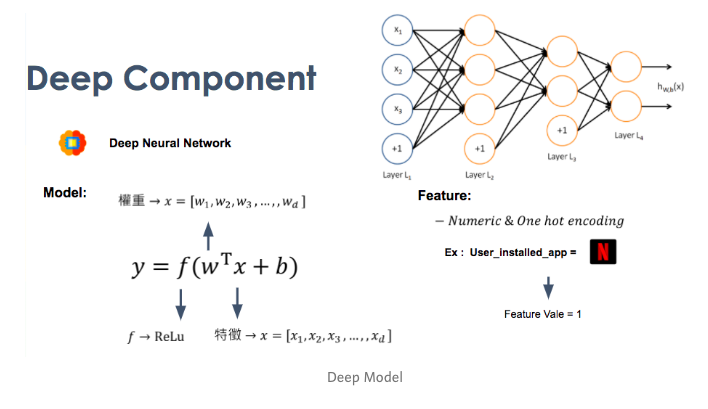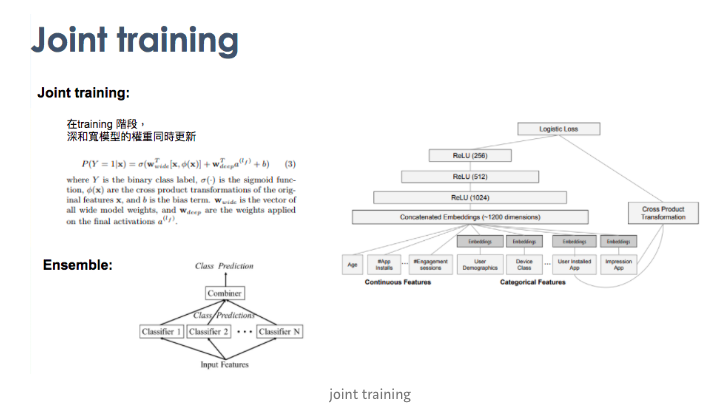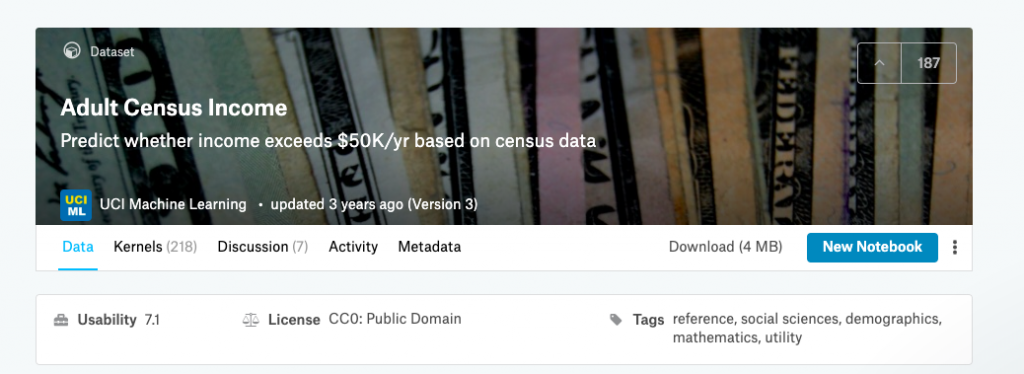``````CSV_COLUMNS = [
"age", "workclass", "fnlwgt", "education", "education_num",
"marital_status", "occupation", "relationship", "race", "gender",
"capital_gain", "capital_loss", "hours_per_week", "native_country",
"income_bracket"
]
``````

Ex : 使用 `tf.feature_column.categorical_column_with_vocabulary_list`

``````gender = tf.feature_column.categorical_column_with_vocabulary_list(
"gender", ["Female", "Male"])
``````

``````occupation = tf.feature_column.categorical_column_with_hash_bucket(
"occupation", hash_bucket_size=1000)
``````

``````crossed_columns = [
tf.feature_column.crossed_column(
["education", "occupation"], hash_bucket_size=1000),
tf.feature_column.crossed_column(
[age_buckets, "education", "occupation"], hash_bucket_size=1000),
tf.feature_column.crossed_column(
["native_country", "occupation"], hash_bucket_size=1000)
]
``````

``````def build_estimator(model_dir, model_type):
"""Build an estimator."""
if model_type == "wide":
m = tf.estimator.LinearClassifier(
model_dir=model_dir, feature_columns=base_columns + crossed_columns)
elif model_type == "deep":
m = tf.estimator.DNNClassifier(
model_dir=model_dir,
feature_columns=deep_columns,
hidden_units=[100, 50])
else:
m = tf.estimator.DNNLinearCombinedClassifier(
model_dir=model_dir,
linear_feature_columns=crossed_columns,
dnn_feature_columns=deep_columns,
dnn_hidden_units=[100, 50])
return m
``````

``````m = build_estimator(model, model_type='wide_n_deep')

train_and_eval('/content/model','wide_n_deep',10,False,False)
``````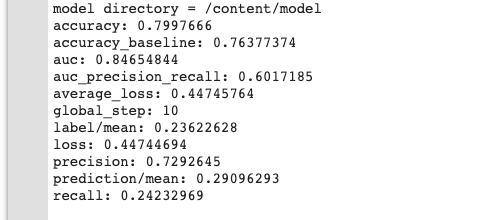### 一天一梗圖:source

### Reference

Wide_n_deep_git

Wide_n_deep_colab